• 在自动控制理论中，典型二阶系统一般是由一个惯性环节和积分环节串联构成：其闭环传递函数为：将上式改为标准形式为:因为实际应用中欠阻尼二阶系统应用最为广泛，这里只分析欠阻尼的情况(0<ξ<1)。欠阻尼系统...


在自动控制理论中，典型二阶系统一般是由一个惯性环节和积分环节串联构成：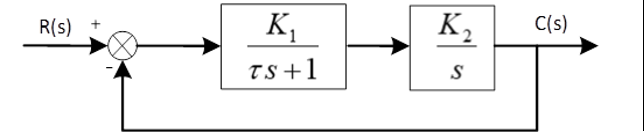其闭环传递函数为：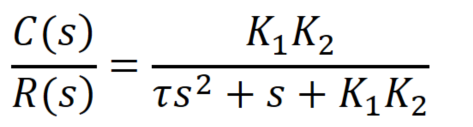将上式改为标准形式为: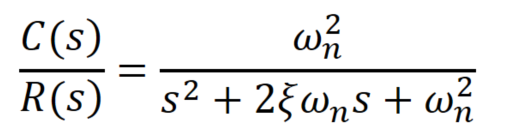因为实际应用中欠阻尼二阶系统应用最为广泛，这里只分析欠阻尼的情况(0<ξ<1)。
欠阻尼系统具有一对共轭复根为: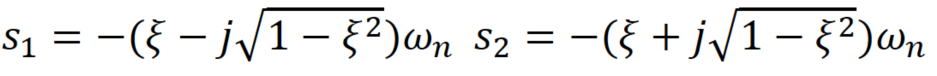其单位阶跃响应的象函数为：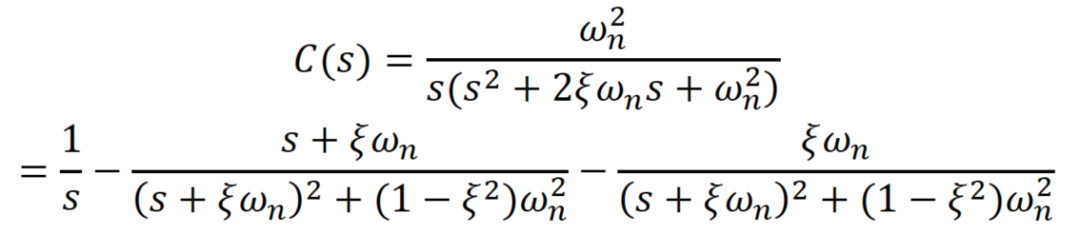经过拉普拉斯反变换可以得到系统单位阶跃响应：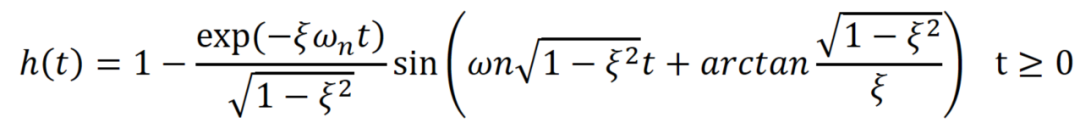下图给出了不同阻尼比时，二阶系统的单位阶跃响应曲线：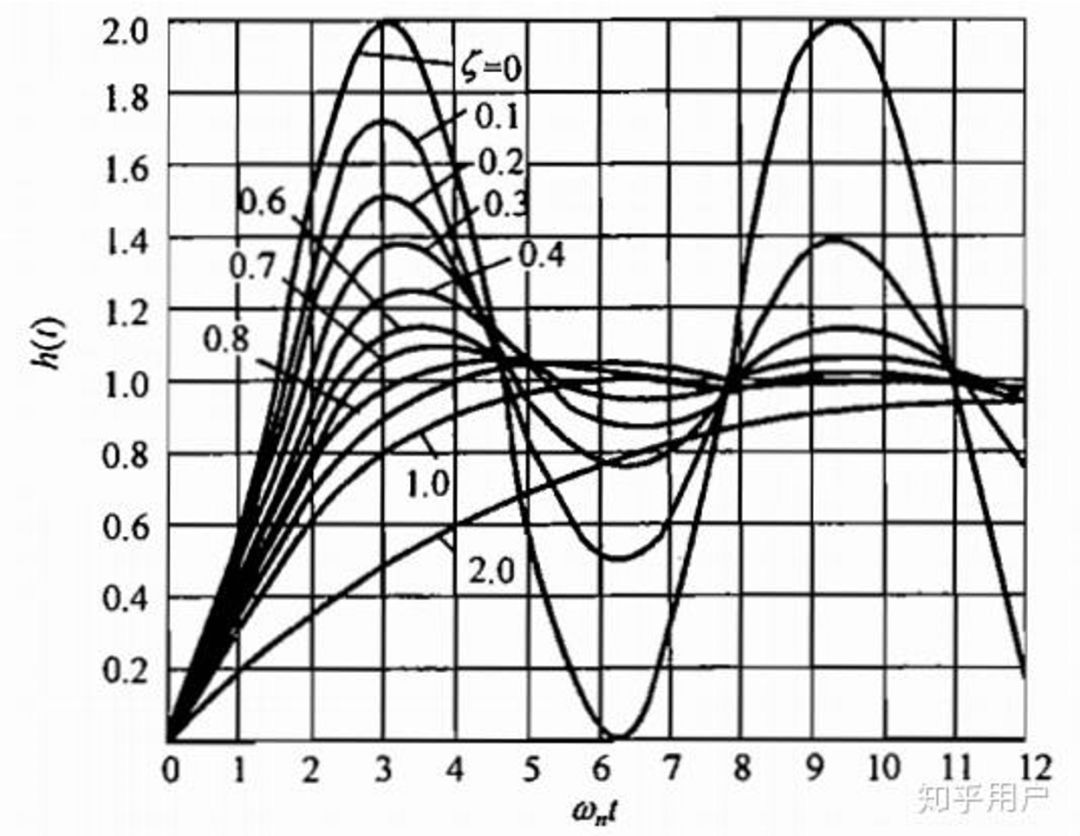根据时域表达式可以得到典型二阶系统单位阶跃响应暂态性能的一些指标：如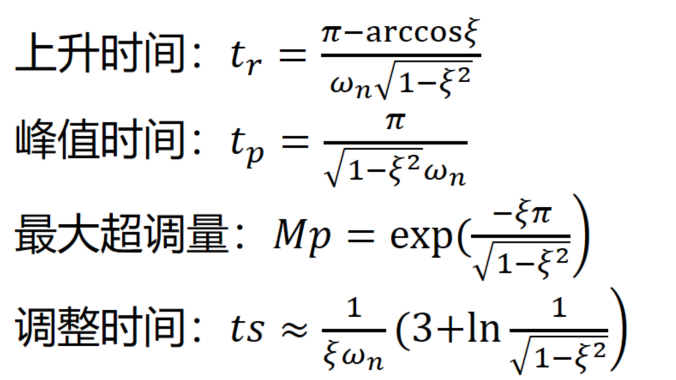调整时间与阻尼比的关系如下图，由图可见，当阻尼比为0.68时，调整时间ts最小，设计二阶系统时，一般取阻尼比为0.707，为最佳阻尼比。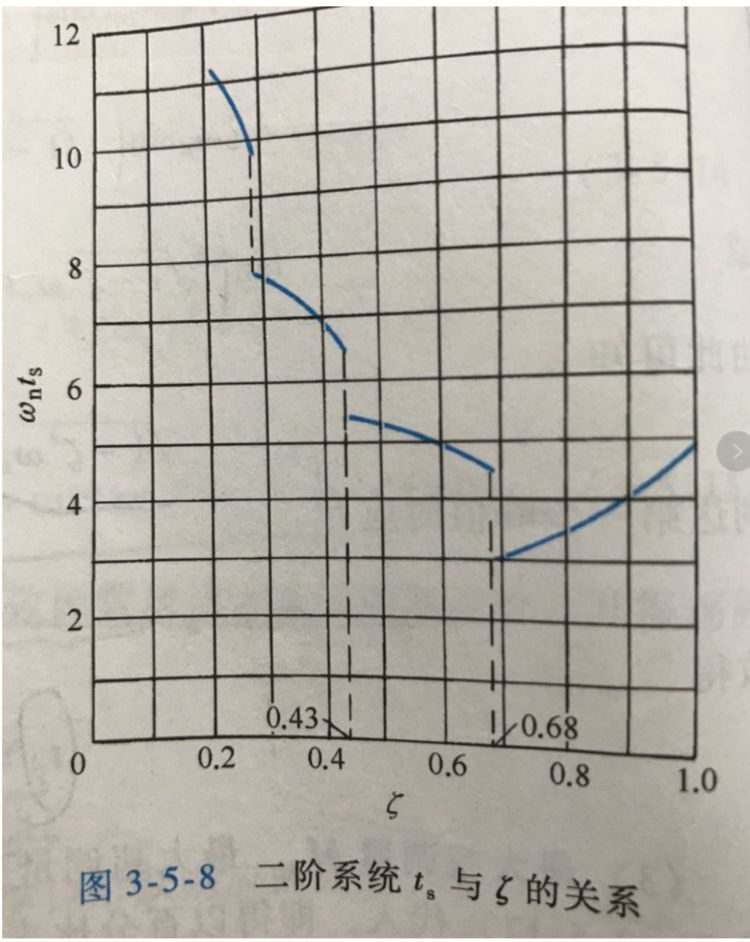以上时典型二阶系统的时域特性，在自动控制理论中都有详细分析，但有时会遇到非典型的二阶系统，它与典型二阶系统关系如何呢？以一种锁相环系统为例：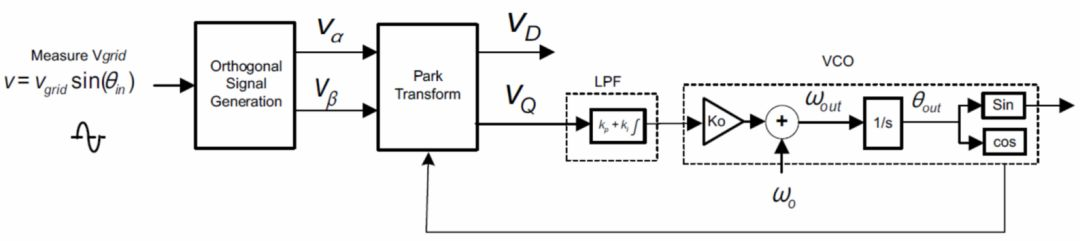上述框图可以简化为：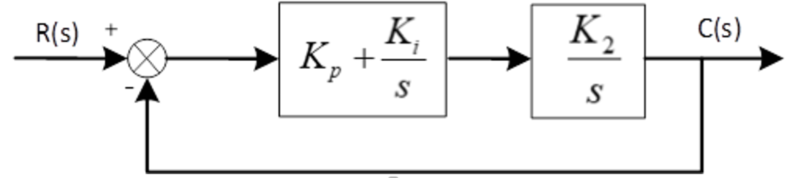同样，上图传递函数写成标准形式为：
可见，与标准形式相比，该二阶系统多了一个零点，而极点相同，按照上述分析方法，可以得到其单位阶跃响应的象函数为：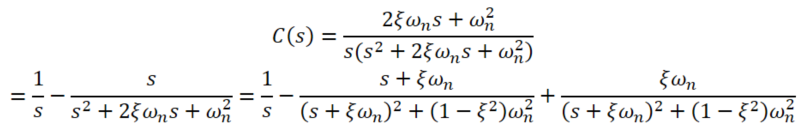可见，与典型二阶系统相比仅第三项的符号有差别，对其进行拉普拉斯反变换得到时域响应表达式：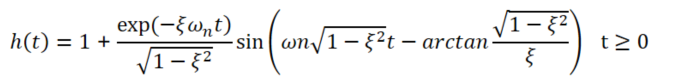与典型二阶系统相比仅第二项符号和相位发生变化，根据时域表达式可以得到典型二阶系统单位阶跃响应暂态性能的一些指标，如下式所示，可以看出一些参数与典型二阶系统有些区别，但相差不大。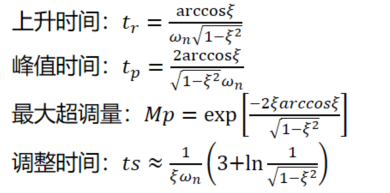为了更明显看出两者之间的差别，令ω=158，在matlab中画出ξ从0.1变为1的阶跃响应曲线，红色为典型二阶系统的响应曲线，蓝色为上文提到的非典型二阶系统的响应曲线，可以看出两者暂态响应相差很小，因此这种情况下典型二阶系统的特性如也可直接应用在文中提到的非典型二阶系统，可以简化设计过程。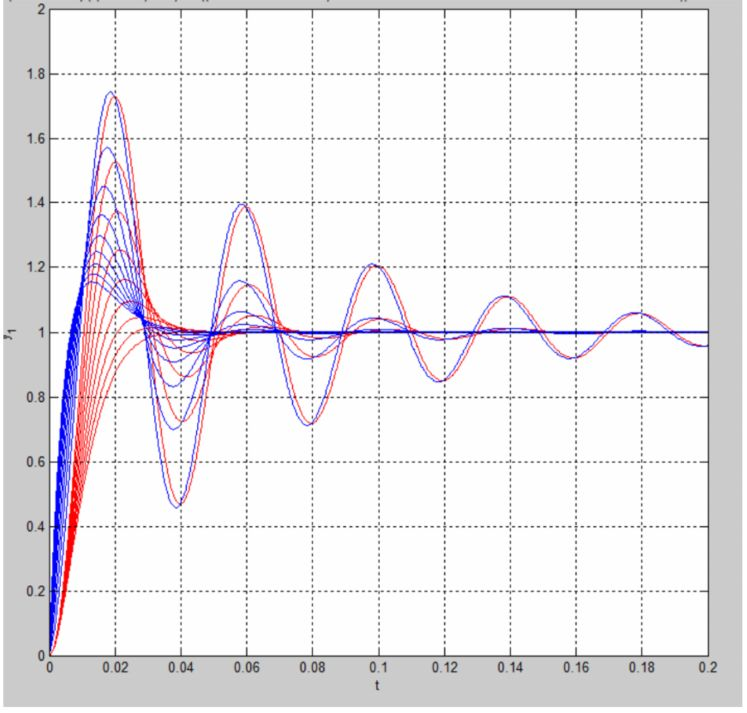其实也可以用根轨迹进行分析，一般远离虚轴且附近无极点的零点，对系统的影响几乎可以忽略。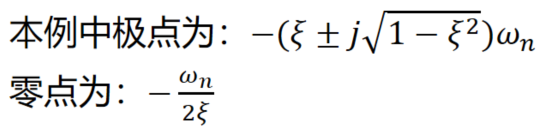显然零点与极点位置相差很远，所以该零点对系统的暂态响应影响不大。
参考文献：
自动控制理论.夏德钤.第四版


展开全文• 二阶系统在不同参数下对单位阶跃信号的响应 一二阶系统 所谓二阶系统就是其输入信号输出信号的关系可用二阶微分方程来表征的系统比如 常见的 RLC 电路图a 单自由度振动系统等 图a 图b 二阶系统传递函数的标准形式为 ...
• 对深入学习二阶系统特性很有帮助，是一个好的资料。
• ## 二阶系统分析

千次阅读 2020-03-10 17:55:43
我在学习自控原理过程中，起初面对二阶系统感到费解，主要原因在于没有实际对照的物理模型和参数物理意义。为此，我专门对二阶系统进行了深入的分析了理解，对其物理含义有了直观的认识，并整理成文与大家分享。 ...
我在学习自控原理过程中，起初面对二阶系统感到费解，主要原因在于没有实际对照的物理模型和参数物理意义。为此，我专门对二阶系统进行了深入的分析了理解，对其物理含义有了直观的认识，并整理成文与大家分享。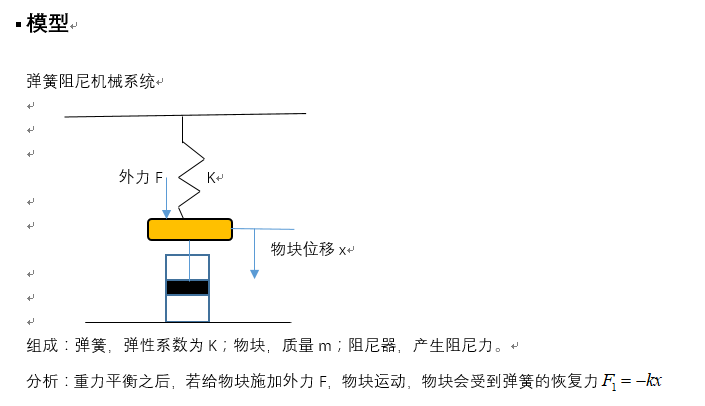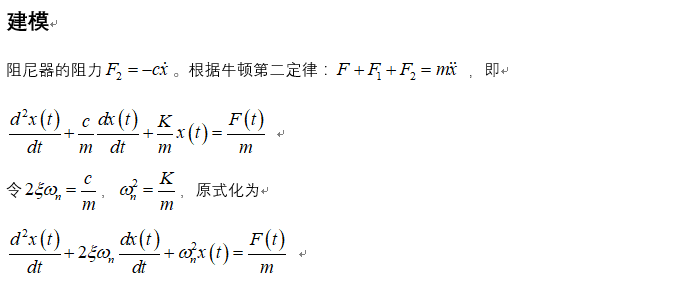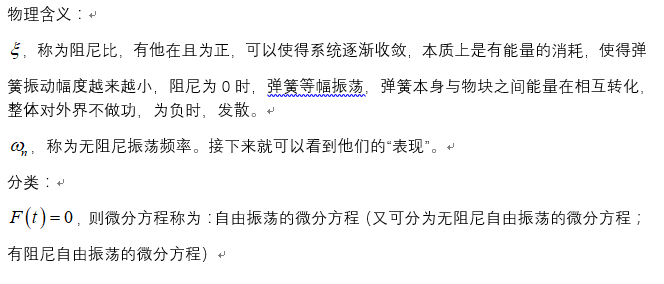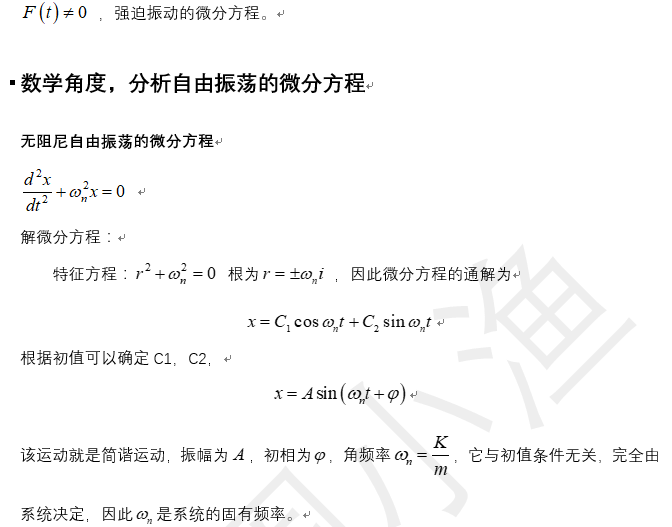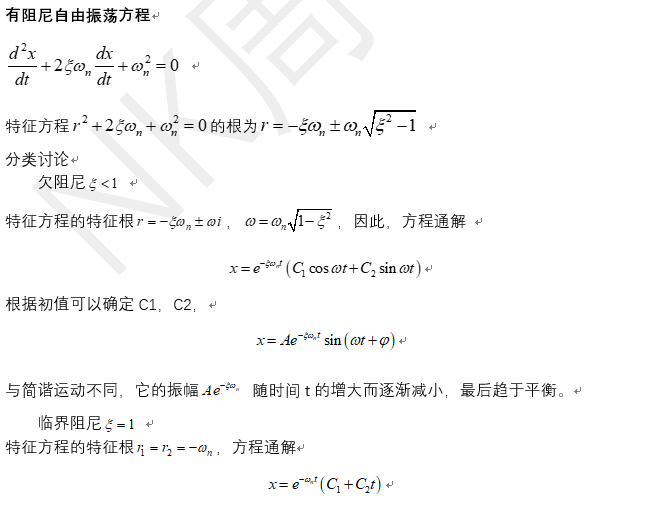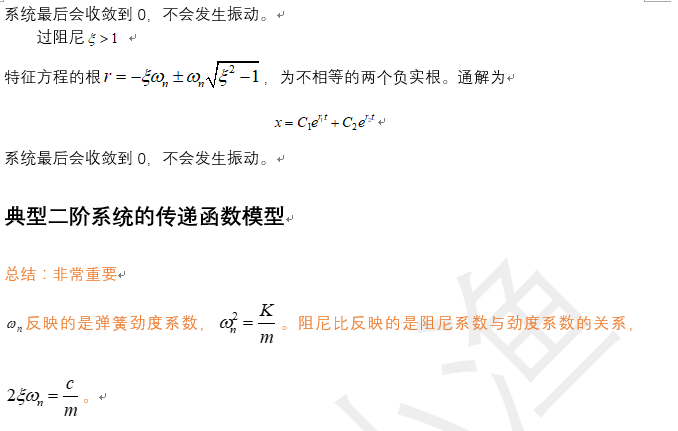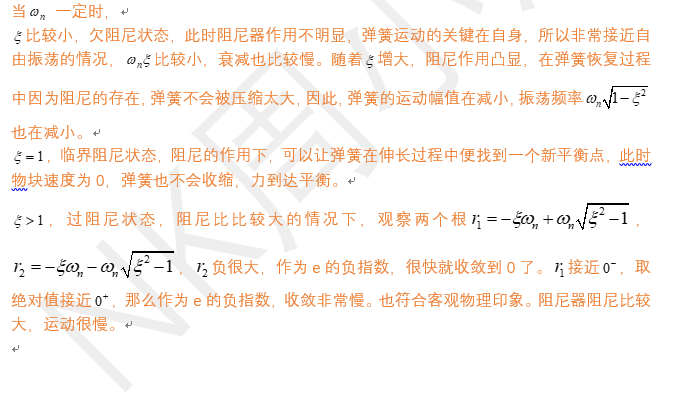展开全文• 二阶系统 所谓二阶系统就是其输入信号输出信号的关系可用二阶微分方程来表征的系统比 如常见的RLC电路图a单自由度振动系统等 LIDIJ LI DI J 1 EQ r 阶系统传递函数的标准形式为 H(s)二 H(s)二 2 ns 二阶系统的Bode图...
• 微分方程形式： 根据克希荷夫定律，电路平衡方程 ur(t)=Ldi(t)dt+Ri(t)+1C∫idtuc=1C∫idt u_r(t)=L\frac{\text{d}i(t)}{\text{d}t}+Ri(t)+\frac{1}{C}\int{}{}i\text{d}t\\ u_c=\frac{1}{C}\int{}{}i\text{d}t ur...


经典数学模型
线性系统

线性系统
R-L-C震荡电路微分方程形式：
根据克希荷夫定律，电路平衡方程

u

r

(

t

)

=

L

d

i

(

t

)

d

t

+

R

i

(

t

)

+

1

C

∫

i

d

t

u

c

=

1

C

∫

i

d

t

u_r(t)=L\frac{\text{d}i(t)}{\text{d}t}+Ri(t)+\frac{1}{C}\int{}{}i\text{d}t\\ u_c=\frac{1}{C}\int{}{}i\text{d}t

消去中间变量

i

(

t

)

i(t)

，整理得：

d

2

u

c

(

t

)

d

t

2

+

R

L

d

u

c

(

t

)

d

t

+

1

L

C

u

c

(

t

)

=

1

L

C

u

r

(

t

)

\frac{\text{d}^2u_c(t)}{\text{d}t^2}+\frac{R}{L}\frac{\text{d}u_c(t)}{\text{d}t}+\frac{1}{LC}u_c(t)=\frac{1}{LC}u_r(t)

传递函数形式：

G

(

s

)

=

U

c

U

r

=

1

L

C

s

2

+

R

L

s

+

1

L

C

=

w

n

2

s

2

+

2

ξ

w

n

s

+

w

n

2

G(s)=\frac{U_c}{U_r}=\frac{\frac{1}{LC}}{s^2+\frac{R}{L}s+\frac{1}{LC}}=\frac{w_n^2}{s^2+2\xi w_ns+w_n^2}

动态方程形式：
动态系统：能储存输入信息（或能量）得系统。
状态变量

x

1

=

i

,

x

2

=

1

C

∫

i

d

t

x_1=i,x_2=\frac{1}{C}\int{}{}i\text{d}t

状态方程为

x

1

˙

=

−

R

L

x

1

−

1

L

x

2

+

1

L

u

r

x

2

˙

=

1

C

x

1

\dot{x_1}=-\frac{R}{L}x_1-\frac{1}{L}x_2+\frac{1}{L}u_r\\ \dot{x_2}=\frac{1}{C}x_1

输出方程

y

=

x

2

y=x_2

整理可得动态方程为：

x

˙

=

A

x

+

B

u

r

y

=

C

x

\dot{\boldsymbol{x}}=\boldsymbol{Ax}+\boldsymbol{Bu_r}\\ y=\boldsymbol{Cx}

其中

A

=

[

−

R

L

−

1

L

1

C

0

]

B

=

[

1

L

0

]

C

=

[

0

1

]

\boldsymbol{A}=\begin{bmatrix} -\frac{R}{L}&-\frac{1}{L}\\ \frac{1}{C}&0 \end{bmatrix} \quad \boldsymbol{B}=\begin{bmatrix} \frac{1}{L}\\0 \end{bmatrix} \quad \boldsymbol{C}=\begin{bmatrix} 0&1 \end{bmatrix}

弹簧-质量-阻尼器系统微分方程形式：

d

2

y

(

t

)

d

t

2

+

f

m

d

y

(

t

)

d

t

+

k

m

y

(

t

)

=

u

(

t

)

m

\frac{\text{d}^2y(t)}{\text{d}t^2}+\frac{f}{m}\frac{\text{d}y(t)}{\text{d}t}+\frac{k}{m}y(t)=\frac{u(t)}{m}

传递函数形式：

G

(

s

)

=

U

Y

=

1

m

s

2

+

f

m

s

+

k

m

=

w

n

2

k

s

2

+

2

ξ

w

n

s

+

w

n

2

G(s)=\frac{U}{Y}=\frac{\frac{1}{m}}{s^2+\frac{f}{m}s+\frac{k}{m}}=\frac{\frac{w_n^2}{k}}{s^2+2\xi w_ns+w_n^2}

动态方程形式：
状态变量

x

1

(

t

)

=

y

(

t

)

,

x

2

=

y

˙

(

t

)

状态方程

x

1

˙

=

x

2

x

2

˙

=

−

k

m

x

1

−

f

m

x

2

+

1

m

u

\dot{x_1}=x_2\\ \dot{x_2}=-\frac{k}{m}x_1-\frac{f}{m}x_2+\frac{1}{m}u

输出方程

y

=

x

2

y=x_2

整理可得动态方程为：

x

˙

=

A

x

+

B

u

r

y

=

C

x

\dot{\boldsymbol{x}}=\boldsymbol{Ax}+\boldsymbol{Bu_r}\\ y=\boldsymbol{Cx}

其中

A

=

[

0

1

−

k

m

−

f

m

]

B

=

[

0

1

m

]

C

=

[

1

0

]

\boldsymbol{A}=\begin{bmatrix} 0&1\\ -\frac{k}{m}&-\frac{f}{m} \end{bmatrix} \quad \boldsymbol{B}=\begin{bmatrix} 0\\\frac{1}{m} \end{bmatrix} \quad \boldsymbol{C}=\begin{bmatrix} 1&0 \end{bmatrix}


展开全文• 控制理论的第一课往往以...1887年，Oliver Heaviside遇到了这个问题，他拿到了类似的微分方程，里面有一阶导数、二阶导数等等，这位大佬看着一阶、二阶这些阶数，突然想到了一个对应关系，如果我们把求一次导看成乘...控制理论的第一课往往以拉普拉斯变换开头，但往往让人困惑的也是这个拉普拉斯变换，为什么要做这样一个变换?先来看个电路的例子：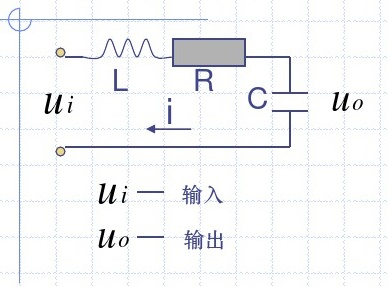由电路知识，可以列写方程：拿到了这个方程，如果没学过微分方程的解法，该怎么办？
1887年，Oliver Heaviside遇到了这个问题，他拿到了类似的微分方程，里面有一阶导数、二阶导数等等，这位大佬看着一阶、二阶这些阶数，突然想到了一个对应关系，如果我们把求一次导看成乘一次数，这不就简单了吗？例如:Oliver随后引入了传递函数的概念，即：他根据工程经验进行了验证，发现这种变换分析电子电路时可以采用。
但问题同样也出现了，这种变换有没有什么数学依据，虽然工程上可以这样变换。
为这种变换找个数学上靠谱的依据不是容易事，1940年左右，有可能是一位数学家的跨行研究导致，人们才意识到，早在1782年，Pierre-Simon-Laplace导出的拉普拉斯变换，早就涵盖了这种方法。
于是，带着探索之心，工程界的众人拿起了数学课本，开始审视拉普拉斯变换。
一个函数的Laplace变换定义为知乎上另一篇文章具体解释了为何能得出Oliver的结果：

李寒潭：【自动控制原理】1.传递函数​zhuanlan.zhihu.com首先，
定义复空间上两个函数的内积

为易证是复空间中的一组正交基。那么根据

内积的意义——一个函数与另一个函数的内积，是这个函数在另一个函数方向上的
投影，可得实函数在复空间基底上的投影为为方便起见，令表示虚变量。（我们后面可以看到它更深层的意义。）则可将该投影式记为（这里的s与实数域中的t相对应，都表示空间上的变量）
同理可证是在实空间中的投影。

有了实空间中与复空间中的一一对应投影关系，我们就可以通过在复空间中对进行分析和运算，从而获知实空间中的性质和运算结果。在做这件事情之前，首先需要对实空间和复空间中的运算关系进行定义。

定义微分算子（这里的define等号表示的是对应关系，而不表示相等）这是因为(设初值为0)

从复空间中微分算子的定义就可以看出选择作为基底的好处了。因为复指数函数有一个最大的优点，就是对它求导等于它自身乘一个数。因此，当我们在实空间中对求一次导数时，在复空间中只需要将它对应的投影式乘以微分算子。这样就极大地简化了求导运算。

类似可定义积分算子为即对进行一次积分，只需对其投影式除以。

拿到了数学上的依据，传递函数获得了自己的根基，正式走入了课本。也拥有了正式的定义
传递函数是指零初始条件下线性时不变系统响应（即输出）量的拉普拉斯变换（或z变换）与激励（即输入）量的拉普拉斯变换之比。
拉普拉斯变换从形式上看比较麻烦，所幸控制工程师无需过于纠结，毕竟有整理好的表格
拉普拉斯变换表 - 百度文库​wenku.baidu.com
除了从微分方程导出传递函数，一些典型环节的传递函数经常用到，有时候还需要处理多个系统连接的情况，需要用到梅森增益公式、结构图化简等技巧。（不作展开）
https://www.bilibili.com/video/av71857275?from=search&seid=5072051921356333345​www.bilibili.com
https://www.bilibili.com/video/av40046656?p=4​www.bilibili.com
（2.3-2.4）
但是，当我们重新审视传递函数定义时，线性时不变系统，这个词让人有点棘手，因为工程里很少有这样的系统，虽然我们可以使用后续的非线性特性来处理，然而在这里，我们可以简单的线性化。线性化的基础是泰勒展开。详见下个链接
[图文]微分方程的线性化 - 百度文库​wenku.baidu.com
另一种方式可以设计补偿环节，使得系统非常接近线性时不变。
参考文献：
1、https://zhuanlan.zhihu.com/p/23617272
2、线性系统理论与设计，Chi-Tsong Chen著
3、控制系统设计指南，George Eills著

System control：控制理论基础（二）时域分析与稳定性​zhuanlan.zhihu.com展开全文• §2.1 二阶常微分方程2.1 Second Order Equations微分方程 MIT公开课《微分方程和线性代数》 2.1 二阶微分方程​v.youku.com二阶常微分方程的应用特别广泛，因为它包含了加速度项，即速度的导数——二阶导数 。...
• 比例—微分控制 ... 自动控制系统在克服误差的调解过程中可能会出现震荡甚至失稳。其原因是由于存在较大惯性环节或有滞后组件，具有抑制误差的作用，其变化总是落后于误差的变化，解决的办法是...
• 1、建模方法：实验法：人为给系统施加某种测试信号，记录相应的输出，然后用适当的数学模型去逼近，也称为系统辨识方法；解析法：利用已有的物理规律和化学规律来分析系统各部分的运动机理，获取其运动方程。2、常用...
• 继上一篇博客 一阶系统时域分析，下面进行二阶系统时域分析，上篇链接：https://blog.csdn.net/qq_40035462/article/details/89350543 前言 在经典控制理论中，常用时域分析法、根轨迹法或频域分析法来分析线性控制...自动控制原理
• 3。2一阶系统的数学模型一阶系统的单位阶跃响应举例说明改善系统性能的简单方法3。...典型二阶系统的瞬态响应线性系统一般不太涉及延迟时间。（二）对于非震荡瞬态过程：通常：需要控制系统有较快的响应...
• 二阶系统在控制工程中比较普遍，且不少高阶系统可化为二阶系统进行分析，故研究和学习二阶系统，具有较大的实际意义。 二阶系统传递函数的标准形式： ...
• 目的是为了分析不同阻尼比对阶跃响应的影响，以及观查二阶系统的频率响应。 二阶系统传递函数如下： 系统框图： （1） 系统阶跃响应，不同阻尼比对应不同的输出。具体参考自动控制原理。当a=1，无超调的...
• 1、二阶系统传递函数的标准形式 典型结构的二阶系统如下图： 其开环传函： 闭环传函： Φ(s)\Phi_{(s)}Φ(s)​为典型二阶系统传递函数的标准形式。ξ\xiξ 为阻尼系数，ωn\omega _{n}ωn​ 为无阻尼震荡自然频率...
• 一、典型二阶系统的参考模拟电路以及Pspice仿真 二、上述模拟线路对应结构框图以及传递函数推导 三、上述系统的时域分析 一、典型二阶系统的参考模拟电路以及Pspice仿真 下图为基于运放的积分线路和比例线路搭建的...matlab
• 单自由度系统考虑一个单自由度二阶系统：叠加法首先介绍一种基于叠加的方法，当看到“叠加”时，基本就可以认定该方法仅适用于线性问题。考虑一个无阻尼单自由度系统在t0时刻受到单位大小的脉冲作用其t时刻的响应为...
• 二阶系统标准形式 Φ(s)=ωn2s2+2ζωns+ωn2 \Phi (s) = \frac{\omega_n^2}{s^2+2\zeta \omega_n s+\omega_n^2} Φ(s)=s2+2ζωn​s+ωn2​ωn2​​ 极点为： s=−ζωn±ωnζ2−1 s=-\zeta \omega_n \pm \omega_n...
• 2）时，系统的输出存在超调量，且决定了超调量的大小，超调量只与有关，和共同决定了系统的响应速度。 越大超调量越小，响应速度越慢。越大系统响应越快。 3）时，系统无超调量，系统的响应速度随的增大而变慢，随...经验分享
• 典型二阶系统的数学模型 R(s) 2 C(s) n s(s 2 ) n : n 无阻尼自振角频率单位是rad/s : 阻尼比相对阻尼系数阻尼系数与临界阻尼系数 的比值 2 n (s) 2 2 s 2 s n n 两种二阶系统的典型形式 R(s) k C(s
• 现在我们看一个单环二阶系统的单位阶跃响应。一个闭环反馈控制系统如下图所示 闭环系统的转换方程为：control
• 2020-05-26-二阶系统时域分析 ...为了使研究结果具有普遍意义，二阶系统的传递函数写为以下标准形式： ωn\omega_{n}ωn​：称为无阻尼自然振荡角频率，简称自然频率。 ωn=KT \omega_{n}=\sqrt{\frac{K}{T}...自动控制原理 笔记
• 上面讲完了最简单的一阶系统动态性能指标，下面说说二阶
• 归一化系统的二阶阶跃响应之matlab GUI+程序打包实现 设计程序效果展示 ...为了研究归一化二阶系统G(s)中阻尼比对单位阶跃响应的影响，于是做了上述1中的效果界面来做可视化分析和展示。G(s)公式如下：其中s...
• 该程序为二阶系统提供了一个 PI 控制器阻尼得到控制，控制器的形式为KP*(1+1/s*TI)。matlab
• 显然这是一个二阶系统，选取状态变量x1x_1x1​为位移，x2x_2x2​为速度，则有： x1˙=x2x2˙=f/m=u\dot{x_1}=x_2\\\dot{x_2}=f/m=ux1​˙​=x2​x2​˙​=f/m=u 其中uuu定义为输入，mmm为物块的质量。又已知： x1(0)...
• 用MATLAB使用ord2命令生成一个标准的二阶系统
• 基于矩阵二阶系统的 Sylvester方程的完全参数化解，通过一组设计参数建立了观测器的所有增益矩阵以及观测器系统矩阵的左特征向量的完全参数化形式。并在此基础上，将H∞范数的上界约束条件转化成一个等价的约束设计...
• 在本研究中，我们首先系统地将一阶近似PML(NPML)技术推广到二阶系统，用谱元法和时域有限差分算法实现。它具有以下优点：通过保持基于二阶PDE的控制方程基本相同，使得实现简单；通过引入一组辅助常微分方程（ODEs）...
• 二阶积分系统极点配置使用二阶积分系统设计的原因： - 二阶积分系统与四旋翼内环具有一定的相似性（动系下的角动量微分需考虑随动） - 二阶积分系统控制器设计相对简单，但包含L1设计需要考虑的全部内容一、二阶...设计 x...